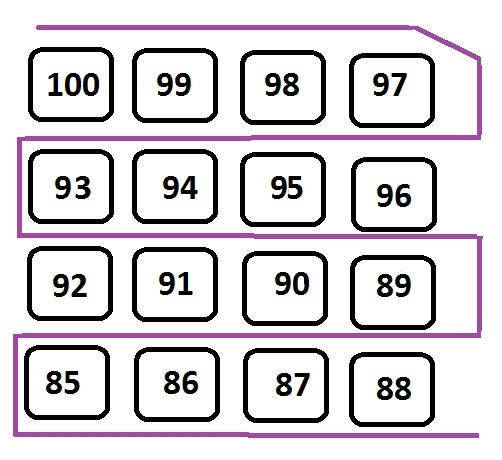# Print matrix in snake pattern in C Programming.

Given an array of nxn size, the program must print the elements of an array in a snake pattern without doing any changes to their original locations## Example

Input: arr[]= 100 99 98 97
93 94 95 96
92 91 90 89
85 86 87 88
Output: 100 99 98 97 96 95 94 93 92 91 90 89 88 87 86 85

The program will traverse each row of a matrix and check for even or odd rows.

• If the row is even it will print the elements of that row from left to right

• If the row is odd it will print the elements of that row from right to left

## Algorithm

START
Step 1 -> create header files for declaring rows and column let’s say of size 4x4
Step 2 -> declare initial variables i and j and array[][] with elements
Step 3 -> Loop For i=0 and i<M and i++
IF i%2==0
Loop For j=0 and j<N and j++
Print arr[i][j]
End
End
Else
Loop For j=N-1 and j>=0 and j—
Print arr[i][j]
End
End
STOP

## Example

Live Demo

#include<stdio.h>
#define M 4
#define N 4
int main() {
int i,j;
int arr[M][N] = {
{ 100, 99, 98, 97 },
{ 93, 94, 95, 96 },
{ 92, 91, 90, 89 },
{ 85, 86, 87, 88 }
};
for (i = 0; i < M; i++) { //for rows
if (i % 2 == 0) {
for (j = 0; j < N; j++) // for column
printf("%d ",arr[i][j]);
} else{
for (j = N - 1; j >= 0; j--)
printf("%d ",arr[i][j]);
}
}
return 0;
}

## Output

if we run the above program then it will generate the following output

100 99 98 97 96 95 94 93 92 91 90 89 88 87 86 85